## 3.3.3 KELDYSH Contour

If one does not consider initial correlations, one can let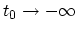. Since we assume that the GREEN's function falls off sufficiently rapidly as a function of the separation of its time arguments, one can neglect the part of the contour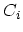extending from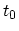to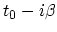. It has been shown that by explicitly taking the initial correlations into account [198,199,200,201] the neglect of this part of the contour corresponds to the neglect of initial correlations. The initial condition, that the system is assumed to be in equilibrium before the external perturbation is turned on, can then be imposed directly on the DYSON equation in integral form. This provides an independent demonstration that, for cases where initial correlations can be neglected, one can discard the contribution of the contour fromto. The contours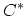and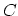become identical, as they both start and end at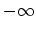. They can be extended beyond the largest time by considering that the time-evolution operator is a unitary, and one then obtains the contour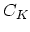introduced by KELDYSH .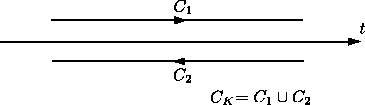M. Pourfath: Numerical Study of Quantum Transport in Carbon Nanotube-Based Transistors